Definitions of Square Dance Calls and Concepts

Index -->  Plus  |  A1  |  A2  |  C1  |  C2  |  C3A  |  C3B  |  C4  |  NOL  |
Definitions (Text Only) -->  Plus  |  A1  |  A2  |  C1  |  C2  |  C3A  |  C3B  |  C4  |  NOL  |
 Find call:

 \$B8@8l(B view (admin)

Initially anyConcept anything anything \$B\$N%3!<%k\$N;O\$a\$N%Q!<%H\$r(B, \$BM?\$(\$i\$l\$?(B CONCEPT \$B\$G9T\$\$\$^\$9!%\$=\$7\$F(B, anything \$B\$N%3!<%k\$N;D\$j\$r9T\$\$\$^\$9(B (\$B\$9\$J\$o\$A(B, \$BM?\$(\$i\$l\$?(B CONCEPT \$B\$r;H\$o\$:\$K(B)\$B!%(B

\$BCm
• anything \$B\$N%3!<%k\$K\$O(B, first part \$B\$,L@3N\$KDj5A\$5\$l\$F\$\$\$kI,MW\$,\$"\$j\$^\$9!%(B
• anything \$B\$N%3!<%k\$N;O\$a\$N%Q!<%H\$r(B, \$BM?\$(\$i\$l\$?(B CONCEPT \$B\$G9T\$C\$?8e(B, \$B7k2L\$N(B formation \$B\$K\$*\$1\$k<+J,\$N0LCV\$r:FI>2A(B (re-evaluate) \$B\$7(B, \$B;D\$j\$N%3!<%k\$rDj5ADL\$j9T\$\$\$^\$9!%(B
• Initially \$B\$O(B meta-concept (\$B%a%?%3%s%;%W%H(B, \$BJL\$N(B concept \$B\$r0z?t\$H\$7\$FMW5a\$9\$k(B concept) \$B\$G\$9!%%3!<%i!<\$O(B Initially anything \$B\$H\$O8@\$(\$^\$;\$s!%(BInitially \$B\$N8@MU\$N8e\$KI,\$:(B concept \$B\$r8@\$o\$J\$1\$l\$P\$J\$j\$^\$;\$s!%(B
\$BLu

•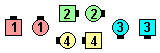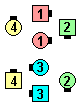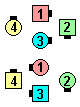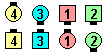\$B\$NA0(B Siamese Diamond Circulate\$B\$N8e(B Very Centers Trade\$B\$N8e(B Centers Cast Off 3/4\$B\$N8e(B (\$B=*\$o\$j(B)
Initially Twice Triple Star Thru :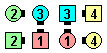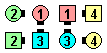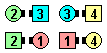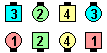\$B\$NA0(B (Centers)Star Thru Twice\$B\$N8e(B (Centers)Mirror Star Thru\$B\$N8e(B Star Thru\$B\$N8e(B (\$B=*\$o\$j(B)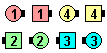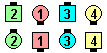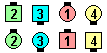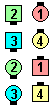\$B\$NA0(B Tandem Hinge\$B\$N8e(B Centers Trade\$B\$N8e(B Step & Fold\$B\$N8e(B (\$B=*\$o\$j(B)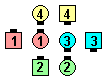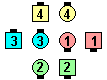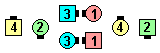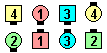\$B\$NA0(B As Couples Scoot Back\$B\$N8e(B Centers Cast Off 3/4 as Outsides Cast Back & Touch 1/2\$B\$N8e(B Centers Counter Rotate 1/4 as Outsides Step & Fold\$B\$N8e(B (\$B=*\$o\$j(B)
Initially Eight By Recoil: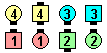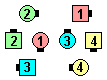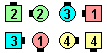\$B\$NA0(B Eight By Box Recycle\$B\$N8e(B Step & Fold\$B\$N8e(B (\$B=*\$o\$j(B)
Initially Checker Box Remake: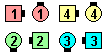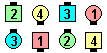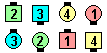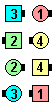\$B\$NA0(B Checker Box Right Arm Turn 1/4\$B\$N8e(B Centers Left Arm Turn 1/2\$B\$N8e(B Right Arm Turn 3/4\$B\$N8e(B (\$B=*\$o\$j(B)

Trade By formation \$B\$+\$i(B, \$B

• Initially Stretched Box Sidetrack:

Centers Pass Thru & all Zig-Zag, all 8 Counter Rotate 1/4, Roll. Double Pass Thru \$B\$G=*\$o\$j\$^\$9!%(B
• Stretched Box Sidetrack:

Centers Pass Thru & all Zig-Zag, Split Counter Rotate 1/4, Roll. \$B8~\$+\$\$9g\$C\$?(B Line \$B\$G=*\$o\$j\$^\$9!%(B

Finally Concept [C3A] (Vic Ceder 1994): \$B;X<(\$5\$l\$?(B anything \$B\$N%3!<%k\$NA4BN\$r9T\$\$\$^\$9\$,(B; \$B;X<(\$5\$l\$?(B CONCEPT \$B\$O(B, \$B;X<(\$5\$l\$?(B anything \$B\$N%3!<%k\$N:G8e\$N%Q!<%H\$@\$1\$K;H\$\$\$^\$9(B.

\$B

• Secondly | Thirdly | Fourthly CONCEPT [C3B]
• Oddly | Evenly CONCEPT [C3B]
• Calls With Parts Calls With Parts (\$B%Q!<%H\$KJ,\$1\$i\$l\$k%3!<%k(B) \$B\$b8+\$F\$/\$@\$5\$\$!%(B
•Choreography for Initially ConceptComments? Questions? Suggestions?

https://www.ceder.net/def/initially.php?level=C3A&language=japan
10-April-2021 16:03:37
Copyright © 2021 Vic Ceder.  All Rights Reserved.